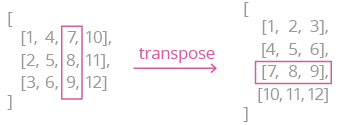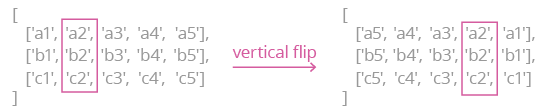Deals Of The Week - hours only!Up to 80% off on all courses and bundles.-Close
Introduction to nested lists
Iterating over nested lists
Modifying nested lists
Working with multiple lists
15. Transposing nested lists
Summary

## Instruction

Very well done! Now, let's tackle a classic programming problem. Suppose we need to transpose a rectangular nested list, i.e. change its rows into columns:How can we do that in Python? Take a look:

numbers_list = [
[1, 4, 7, 10],
[2, 5, 8, 11],
[3, 6, 9, 12]
]

list_to_return = []
for i in range(len(numbers_list)):
new_row = []
for j in range(len(numbers_list)):
new_row.append(numbers_list[j][i])
list_to_return.append(new_row)

Note what happens:

1. In the outer loop (i), we iterate over the length of the first nested list, i.e. over the "columns".
2. Inside the loop, we create a variable for a new row (an empty list).
3. In the inner loop (j), we iterate over the outer list's length, i.e. over the "rows".
4. Inside the inner loop, we add the i-th element from each nested list:
5. We add the new row to the new list.

## Exercise

Write a function named vertical_flip(input_list) that accepts a 2D list and flips it vertically:Do not return a copy of the list. Instead, modify the original one.

### Stuck? Here's a hint!

Use the following nested for loops:

for i in range(len(input_list)):
for j in range(len(input_list[i]) // 2):

Inside the nested loop, swap two elements:

input_list[i][j]
and
input_list[i][len(input_list[i]) - j - 1]